Processing ......FreeComputerBooks.com Links to Free Computer, Mathematics, Technical Books all over the World

Introduction to Probability, Statistics, and Random Processes
Top Free C Programming Books 🌠 - 100% Free or Open Source!
• Title: Introduction to Probability, Statistics, and Random Processes
• Author(s) Hossein Pishro-Nik
• Publisher: Kappa Research, LLC (August 24, 2014)
• Hardcover: 744 pages
• eBook: HTML and PDF Files
• Language: English
• ISBN-10/ASIN: 0990637204
• ISBN-13: 978-0990637202Book Description

This book introduces students to probability, statistics, and stochastic processes. It can be used by both students and practitioners in engineering, various sciences, finance, and other related fields. It provides a clear and intuitive approach to these topics while maintaining mathematical accuracy.

• Basic concepts such as random experiments, probability axioms, conditional probability, and counting methods
• Single and multiple random variables (discrete, continuous, and mixed), as well as moment-generating functions, characteristic functions, random vectors, and inequalities
• Limit theorems and convergence
• Introduction to Bayesian and classical statistics
• Random processes including processing of random signals, Poisson processes, discrete-time and continuous-time Markov chains, and Brownian motion
• Simulation using MATLAB and R (online chapters)

The book contains a large number of solved exercises. The dependency between different sections of this book has been kept to a minimum in order to provide maximum flexibility to instructors and to make the book easy to read for students. Examples of applications such as engineering, finance, everyday life, etc. are included to aid in motivating the subject.

• N/A
Reviews, Ratings, and Recommendations: Related Book Categories: Read and Download Links:Similar Books:
•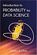Introduction to Probability for Data Science (Stanley Chan)

This book is an introductory textbook in undergraduate probability in the context of data science to emphasize the inseparability between data (computing) and probability (theory) in our time, with examples in both MATLAB and Python.

•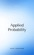Applied Probability (Paul Pfeiffer)

This book presents a unique blend of theory and applications, with special emphasis on mathematical modeling, computational techniques, and examples from the real world application in industries and science.

•Probability Theory: The Logic of Science (E. T. Jaynes)

Going beyond the conventional mathematics of probability theory, this study views the subject in a wider context. It discusses new results, along with applications of probability theory to a variety of problems.

•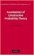Foundations of Constructive Probability Theory (Yuen-Kwok Chan)

This book provides a systematic and general theory of probability within the framework of Constructive Mathematics. It can serve as a parallel introduction into constructive mathematics and rigorous probability theory.

•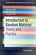Introduction to Random Matrices: Theory and Practice

This is a book for absolute beginners. The aim is to provide a truly accessible introductory account of Random Matrix theory. Most chapters are accompanied by MATLAB codes to guide readers through the numerical check of most analytical results.

•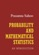Probability and Mathematical Statistics (Prasanna Sahoo)

This book presents an introduction to probability and mathematical statistics for students already having some elementary mathematical background. It blends proven coverage with new innovations to ensure you gain a solid understanding of statistics.

•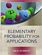Elementary Probability for Applications (Rick Durrett)

This clear and lively introduction to probability theory concentrates on the results that are the most useful for applications, including combinatorial probability and Markov chains. Concise and focused, it is designed for students familiar with basic calculus.

•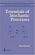Essentials of Stochastic Processes (Rick Durrett)

Stochastic processes have become important for many fields, including mathematical finance and engineering. Written by one of the worlds leading probabilists, this book presents recent results previously available only in specialized monographs.

•Introduction to Probability and Statistics Using R (G. Jay Kerns)

This is a textbook for an undergraduate course in probability and statistics. The approximate prerequisites are calculus and some linear algebra. Students attending the class include mathematics, engineering, and computer science majors.

Book Categories
 :All CategoriesTop Free BooksRecent BooksMiscellaneous BooksComputer EngineeringComputer LanguagesComputer ScienceData Science/DatabasesJava and Java EE (J2EE)Linux and UnixMathematicsMicrosoft and .NETMobile ComputingNetworking and CommunicationsSoftware EngineeringSpecial TopicsWeb Programming
Other Categories